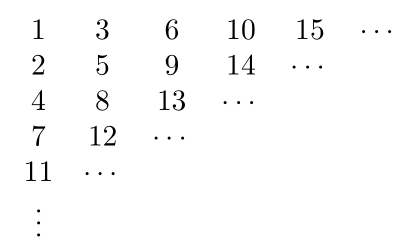If you find any mistakes, please make a comment! Thank you.

## Solution to Understanding Analysis Exercise 1.2

##### Exercise 1.2.1

See Understanding Analysis Instructors’ Solution Manual Exercise 1.2.1

##### Exercise 1.2.2

Suppose there exists a rational number $r$ such that $2^r=3$. Let $r=p/q$, where $p$ and $q$ are integers. Then$2^{p/q}=3\Longrightarrow 2^p=3^q.$Note that $q\ne 0$.

If $q>0$, then $p>0$. Hence we have $3|2^p$, which is impossible.

If $q<0$, then $p<0$. We have $2^{-p}=3^{-q}$. Similarly, we have $3|2^{-p}$, which is impossible. Thus completing the proof.

##### Exercise 1.2.3

See Understanding Analysis Instructors’ Solution Manual Exercise 1.2.2

##### Exercise 1.2.4

Rearrange $\mathbf N$ as follows,Take $A_i$ as the set of numbers in $i$-th column. Then it is clear that $A_i\cap A_j=\emptyset$ for $i\ne j$ and $\cup_{i=1}^\infty A_i=\mathbf N$.

##### Exercise 1.2.5

(a) If $x\in (A\cap B)^c$, hence $x\notin A\cap B$. Therefore, we have $x\notin A$ or $x\notin B$ which implies that $x\in A^c$ or $x\in B^c$. Thus $x\in A^c\cup B^c$ and hence $(A\cap B)^c\subseteq A^c\cup B^c$.

(b) If $x\in A^c \cup B^c$, then $x\in A^c$ or $x\in B^c$. Hence $x\notin A$ or $x\notin B$, namely $x\notin A\cap B$. Thus $x\in (A\cap B)^c$. Therefore, we have $A^c\cup B^c\subseteq (A\cap B)^c$.

(c) If $x\in (A\cup B)^c$, then $x\notin A\cup B$. Hence $x\notin A$ and $x\notin B$, namely $x\in A^c$ and $x\in B^2$. Thus $x\in A^c\cap B^c$. We conclude that $(A\cup B)^c\subseteq A^c\cap B^c$.

If $x\in A^c\cap B^c$, Then $x\in A^c$ and $x\in B^c$, namely $x\notin A$ and $x\notin B$. Therefore $x\notin A\cup B$ and thus $x\in (A\cup B)^c$. We conclude that $A^c\cap B^c\subseteq (A\cup B)^c$.

As a consequence, $(A\cup B)^c=A^c \cap B^c$.

##### Exercise 1.2.6

(a) If $a$ and $b$ are both non-negative, then $|a+b|=a+b$, $|a|=a$, and $|b|=b$. Hence$|a+b|=a+b=|a|+|b|.$If $a$ and $b$ are both non-positive, then $|a+b|=-(a+b)$, $|a|=-a$, and $|b|=-b$. Hence$|a+b|=-(a+b)=-a-b=|a|+|b|.$

(b) Note that $$(a+b)^2=a^2+2ab+b^2$$ and $$(|a|+|b|)^2=|a|^2+2|a||b|+|b|^2=a^2+2|a||b|+b^2.$$ Since $ab\leqslant |a b|$, we have $$(a+b)^2\leqslant (|a|+|b|)^2.$$Since $x^2\leqslant y^2$ implies $|x|\leqslant |y|$, we have $$|a+b|\leqslant |a|+|b|.$$

(c) We applies the triangle inequality several times, \begin{align*}&~|a-c|+|c-d|+|d-b|\\ \geqslant &~|(a-c)+(c-d)|+|d-b|\\ =&~|a-d|+|d-b|\geqslant |(a-d)+(d-b)|\\ =&~|a-b|.\end{align*}

(d) Note that $$||a|-|b||^2=(|a|-|b|)^2=a^2-2|a||b|+b^2$$ and $$|a-b|^2=(a-b)^2=a^2-2ab+b^2.$$Since $ab\leqslant |a||b|$, we have $-2|a||b|\leqslant 2ab$. Hence\begin{align*}||a|-|b||^2=&~(|a|-|b|)^2=a^2-2|a||b|+b^2\\ \leqslant & ~a^2-2ab+b^2=|a-b|^2.\end{align*}Therefore $$||a|-|b||\leqslant |a-b|.$$

##### Exercise 1.2.7

See Understanding Analysis Instructors’ Solution Manual Exercise 1.2.6

##### Exercise 1.2.8

(a) $f(n)=n+1$ for $n\in\mathbf N$.

(b) $f(1)=1$ and $f(n)=n-1$ for $n\geqslant 2$.

(c) $f(1)=0$, $f(2n)=n$ and $f(2n+1)=-n$ for all $n\mathbf N$.

##### Exercise 1.2.9

See Understanding Analysis Instructors’ Solution Manual Exercise 1.2.7

##### Exercise 1.2.10

(a) & (b) False. Take $a=b=0$ as a counterexample.

(c) True. Let us prove it. Consider the “if” part. Suppose $a < b$, then we are done. Suppose $a\geqslant b$, then$|a-b|=a-b.$By assumption, $|a-b|=a-b < \varepsilon$ for every $\varepsilon>0$. By Theorem 1.2.6, we have $a=b$. Hence we always have $a\leqslant b$.

“Only if” part. If $a\leqslant b$, then it is clear that$a< b+\varepsilon$for every $\varepsilon>0$.

##### Exercise 1.2.11

(a) True. When $n$ is very large, then $\dfrac{1}{n}$ is very small and close to zero.

(b) False. See above. We cannot find a positive number which is small than any positive number.

(c) True. See Theorem 1.4.3.

##### Exercise 1.2.12

(a) It is clear $y_1=6> -6$. Now we suppose that $y_n> -6$. Now we show that $y_{n+1}> -6$. Since $y_{n+1}=\dfrac{2y_n-6}{3}$, we have\begin{align*}y_{n+1}=&~\dfrac{2y_n-6}{3}\\ >&~\frac{2\times (-6)-6}{3}=\frac{-18}{3}=-6.\end{align*}By induction, we have $y_n> -6$ for all $n\in\mathbf N$.

(b)It is equivalent to show that $y_n> y_{n+1}$ for all $n\in\mathbf N$. Note that $y_2=\dfrac{2\cdot 6-6}{3}=2$, hence we have $y_1 > y_2.$Now we suppose that $y_n > y_{n+1}$, namely $y_n-y_{n+1}< 0$, we would like to show that $y_{n+1}> y_{n+2}$. Since $y_{n+1}=\dfrac{2y_n-6}{3}$, we have\begin{align*}y_{n+2}-y_{n+1}=&~\frac{2y_{n+1}-6}{3}-\frac{2y_n-6}{3}\\ =&~\frac{2(y_{n+1}-y_n)}{3}< 0.\end{align*}Hence $y_{n+2}< y_{n+1}$. By induction, we have $y_n> y_{n+1}$ for all $n\in\mathbf N$, which implies that the sequence $(y_1,y_2,\cdots)$ is decreasing.

##### Exercise 1.2.13

See Understanding Analysis Instructors’ Solution Manual Exercise 1.2.12